# JAC Class 10 Maths Solutions Chapter 7 Coordinate Geometry Ex 7.2

Jharkhand Board JAC Class 10 Maths Solutions Chapter 7 Coordinate Geometry Ex 7.2 Textbook Exercise Questions and Answers.

## JAC Board Class 10 Maths Solutions Chapter 7 Coordinate Geometry Exercise 7.2

Question 1.
Find the coordinates of the point which divides the join of (- 1, 7) and (4, – 3) in the ratio 2 : 3.
Let P(x, y) be the required point which divides the join of (- 1, 7) and (4, – 3) in the ratio 2 : 3.
Then, using the section formula, we get
x = $$\frac{2(4)+3(-1)}{2+3}$$ = $$\frac{8-3}{5}$$ = 1 and
y = $$\frac{2(-3)+3(7)}{2+3}$$ = $$\frac{-6+21}{5}$$ = 3
Thus, the coordinates of the point which divides the join of (- 1, 7) and (4, – 3) in the ratio 2 : 3 are (1, 3).Question 2.
Find the coordinates of the points of trisection of the line segment joining (4, – 1) and (- 2, – 3).
Let A (4, – 1) and B (- 2, – 3) be the given points and let P and Q be the points of trisection of AB.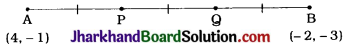Then, AP = PQ = QB
Hence, P divides AB in the ratio 1 : 2.
Therefore, the coordinates of P, by applying the section formula, are
($$\frac{1(-2)+2(4)}{1+2}, \frac{1(-3)+2(-1)}{1+2}$$) = (2, – $$\frac{5}{3}$$)
Now, Q divides AB in the ratio 2 : 1.
Therefore, the coordinates of Q, by applying the section formula, are
($$\frac{2(-2)+1(4)}{2+1}, \frac{2(-3)+1(-1)}{2+1}$$) = (0, – $$\frac{7}{3}$$)
Thus, the coordinates of the points of trisection of the line segment joining (4, – 1) and (- 2, – 3) are (2, – $$\frac{5}{3}$$) and (0, – $$\frac{7}{3}$$).

Alternative method for Q :
Here, Q is the midpoint of the line segment joining P(2, – $$\frac{5}{3}$$) and B(- 2, – 3). Therefore, by applying the midpoint formula, the coordinates of Q are
($$\frac{2+(-2)}{2}$$, $$\frac{-\frac{5}{3}+(-3)}{2}$$) = (0, – $$\frac{7}{3}$$)

Question 3.
To conduct Sports Day activities, in your rectangular shaped school ground ABCD, lines have been drawn with chalk powder at a distance of 1m each. 100 flower pots have been placed at a distance of 1 m from each other along AD, as shown in the given figure. Niharika runs $$\frac{1}{4}$$th the distance AD on the 2nd line and posts a green flag. Preet runs $$\frac{1}{5}$$th the distance AD on the eighth line and posts a red flag. What is the distance between both the flags? If Rashmi has to post a blue flag exactly halfway between the line segment joining the two flags, where should she post her flag?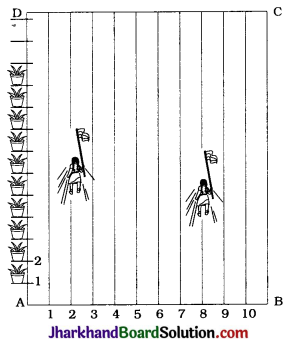Let us consider rectangle ABCD as coordinate plane with A as the origin.
Then, AD = 100 m and each lane number will indicate its distance on the x-axis. Niharika $$\frac{1}{4}$$th the distance AD.
∴ Distance covered by Niharika = $$\frac{1}{4}$$ × 100 m
= 25 m
Niharika runs on the second line.

Hence, the coordinates of the point N where Niharika posts the green flag are (2, 25). Following the same notations, the coordinates of the point P where Preet posts the red flag are (8, 20).
To find the distance (in metres) between the two flags, we have to find the length of join of N (2, 25) and P(8, 20).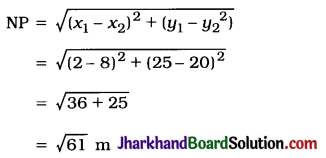Now, Rashmi has to post a blue flag exactly halfway between the green flag and the red flag.
So, we have to find the midpoint R of the join of N (2, 25) and P(8, 20).

Then, the coordinates of R, using the midpoint formula, are
($$\frac{2+8}{2}, \frac{25+20}{2}$$) = (5, 22.5)
This suggest that Rashmi should post the blue flag on the fifth line at distance of 22.5 m from AB.
Thus, the distance between the green flag and the red flag is $$\sqrt{61}$$m and Rashmi should post the blue flag on the 5th line at distance 22.5 m from AB.Question 4.
Find the ratio in which the line segment joining the points (- 3, 10) and (6, – 8) is divided by (- 1, 6).
Let P(- 1, 6) divide internally the line segment joining the points A(- 3, 10) and B (6, – 8) in the ratio m1 : m1.
Using the section formula, we get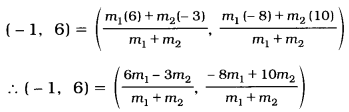Comparing the x-coordinates on both the sides, we get
∴ – 1 = $$\frac{6 m_1-3 m_2}{m_1+m_2}$$
∴ – m1 – m< sub>2 = 6m1 – 3m2
∴ 7m1 = 2m2
∴ $$\frac{m_1}{m_2}=\frac{2}{7}$$
∴ m1 : m2 = 2 : 7
Then, to verify the y-coordinate,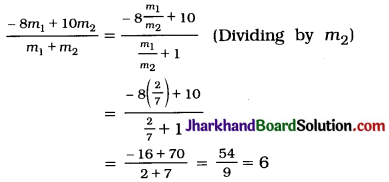Thus, the point (- 1, 6) divides the line segment joining the points (- 3, 10) and (6, – 8) in the ratio 2 : 7.

Question 5.
Find the ratio in which the line segment joining A(1, 5) and B (- 4, 5) is divided by the x-axis. Also find the coordinates of the point of division.
Let the ratio in which the line segment joining A(1, – 5) and B(- 4, 5) is divided by the x-axis be k : 1.
Then, by the section formula, the coordinates of the point which divides AB in the ratio k : 1 are
($$\frac{k(-4)+1(1)}{k+1}, \frac{k(5)+1(-5)}{k+1}$$), i.e. ($$\frac{1-4 k}{k+1}$$, $$\frac{5 k-5}{k+1}$$)
Now, the point of division is on the x-axis.
Hence, its y-coordinate is 0.
∴ $$\frac{5 k-5}{k+1}$$ = 0
∴ k = 1
Hence, the required ratio k : 1 is 1 : 1.
Now, the coordinates of the point of division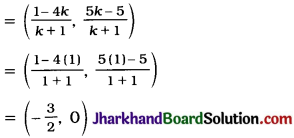Thus, the x-axis divides the line segment joining A(1, – 5) and B(- 4, 5) in the ratio 1 : 1 at the point (-$$\frac{3}{2}$$, 0).Question 6.
If (1, 2), (4, y), (x, 6) and (3, 5) are the vertices of a parallelogram taken in order, find x and y.
Let A(1, 2), B(4, y), C(x, 6) and D(3, 5) be the vertices of parallelogram ABCD. As ABCD is a parallelogram, its diagonals AC and BD bisect each other.
∴ Midpoint of AC = Midpoint of BD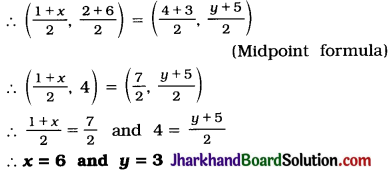Question 7.
Find the coordinates of a point A, where. AB is the diameter of a circle whose centre is (2, – 3) and B is (1, 4).
Let the coordinates of A be (x, y).
In a circle, centre is the midpoint of every diameter.
Here, AB is a diameter and (2, – 3) is the centre.
∴ Midpoint of AB = (2, – 3)
∴ ($$\frac{x+1}{2}, \frac{y+4}{2}$$) = (2, – 3)
∴ $$\frac{x+1}{2}$$ = 2 and $$\frac{y+4}{2}$$ = – 3
∴ x = 3 and y = – 10
Thus, the coordinates of A are (3, -10).

Question 8.
If A and B are (- 2, – 2) and (2, 4). respectively, find the coordinates of P such that AP = $$\frac{3}{7}$$AB and P lies on the line segment AB.
A(- 2, – 2) and B (2, – 4) are given points and point P(x, y) lies on line segment AB such that AP = $$\frac{3}{7}$$AB.
Then, PB = AB – AP = AB – $$\frac{3}{7}$$AB = $$\frac{4}{7}$$AB.
Now, $$\frac{AP}{PB}$$ = $$\frac{\frac{3}{7} \mathrm{AB}}{\frac{4}{7} \mathrm{AB}}$$ = $$\frac{3}{4}$$
Thus, P(x, y) divides AB in the ratio 3 : 4.
Then, by section formula
(x, y) = ($$\frac{3(2)+4(-2)}{3+4}, \frac{3(-4)+4(-2)}{3+4}$$)
∴ (x, y) = (-$$\frac{2}{7}$$, – $$\frac{20}{7}$$)
Thus, the coordinates of P are (-$$\frac{2}{7}$$, – $$\frac{20}{7}$$)

Question 9.
Find the coordinates of the points which divide the line segment joining A(-2, 2) and B (2, 8) into four equal parts.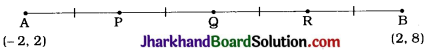Let P, Q and R be the points which divide the line segment AB joining A(-2, 2) and B(2, 8) into four equal parts.
Then, Q is the midpoint of AB.
Hence, by midpoint formula, the coordinates of Q are
($$\frac{-2+2}{2}, \frac{2+8}{2}$$) = (0, 5)
Now, P is the midpoint of Ag.
Hence, by midpoint formula, the coordinates of P are
($$\frac{-2+0}{2}, \frac{2+5}{2}$$) = (-1, $$\frac{7}{2}$$)
Again, R is the midpoint of QB.
Hence, by midpoint formula, the coordinates of R are
($$\frac{0+2}{2}, \frac{5+8}{2}$$) = (1, $$\frac{13}{2}$$)
Thus, the coordinates of the required points are (- 1, $$\frac{7}{2}$$), (0, 5) and (1, $$\frac{13}{2}$$).Question 10.
Find the area of a rhombus if its vertices are (3, 0), (4, 5), (- 1, 4) and (- 2, – 1) taken in order.
[Hint: Area of a rhombus = $$\frac{1}{2}$$ (product of its diagonals)]
Diagonal AC = $$\sqrt{(3+1)^2+(0-4)^2}$$
= $$\sqrt{32}$$ = 4 $$\sqrt{2}$$ units
Diagonal BD = $$\sqrt{(4+2)^2+(5+1)^2}$$
= $$\sqrt{72}$$ = 6$$\sqrt{2}$$ units
= $$\frac{1}{2}$$(Products of its diagonals)
∴ Area of rhombus ABCD = $$\frac{1}{2}$$ × AC × BD
= $$\frac{1}{2}$$ × 4$$\sqrt{2}$$ × 6$$\sqrt{2}$$